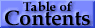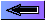### 1.   Introduction

This article outlines the main concepts of atomic structure, with some emphasis on terminology and notation. Atomic radiation is discussed, in particular the wavelengths, intensities, and shapes of spectral lines, and a few remarks are made regarding continuous spectra. We include updated tabulations of ionization energies for the neutral atoms and transition probabilities for persistent lines of selected neutral atoms. Some sources of additional atomic spectroscopic data are mentioned.

Experimental techniques and the details of atomic theoretical methods are not covered in this article; these and a number of other subjects pertinent to atomic spectroscopy are treated in one or more of at least fifteen chapters in the volume Atomic, Molecular, and Optical Physics Handbook, G.W.F. Drake, Ed. (AIP Press, Woodbury, NY, 1996) and in references below.

### 2.   Frequency, Wavenumber, Wavelength

The photon energy due to an electron transition between an upper atomic level k (of energy Ek) and a lower level i is
 ΔE = Ek - Ei = hν = hcσ = hc/λvac  , (1)
where ν is the frequency, σ the wavenumber in vacuum, and λvac the wavelength in vacuum. The most accurate spectroscopic measurements are determinations of transition frequencies, the unit being the Hertz (1 Hz = cycle/sec) or one of its multiples. A measurement of any one of the entities frequency, wavenumber, or wavelength (in vacuum) is an equally accurate determination of the others since the speed of light is exactly defined. The most common wavelength units are the nanometer (nm), the Ångström (1 = 10-1 nm) and the micrometer (µm). The SI wavenumber unit is the inverse meter, but in practice wavenumbers are usually expressed in inverse centimeters: 1 cm-1 = 102 m-1, equivalent to 2.997 924 58 × 104 MHz.

In addition to frequency and wavenumber units, atomic energies are often expressed in electron volts (eV). One eV is the energy associated with each of the following quantities:

 2.417 989 40(21) × 1014 Hz 8 065.544 45(69) cm-1 1 239.841 91(11) nm  11 604.505(20) K (kelvin)  1.602 176 53(14) × 10-19 J (joule)
We note that the basic unit of temperature, the kelvin, is equivalent to about 0.7 cm-1, i.e., the value of the Boltzmann constant k expressed in wavenumber units per kelvin is 0.695 035 6(12) cm-1/K. One reason for citing this particular equivalency involving the internationally accepted symbol for the kelvin (K)  is to suggest that use of the letter K as a symbol for 1 cm-1 (1 kayser) should be discontinued.

The unit of energy in the system of atomic units (a.u.) often used for theoretical calculations is the hartree, which is equal to 2 rydbergs. The rydberg for an atom having nuclear mass M is

 1 Ry = RM = M(M + me)-1 R∞  , (2)
with
 R∞ = mecα2/(2h) = 10 973 731.568 525(73) m-1  , (3)

which is equivalent to 13.605 692 3(12) eV. The Rydberg constant R is thus the limit value for infinite nuclear mass.